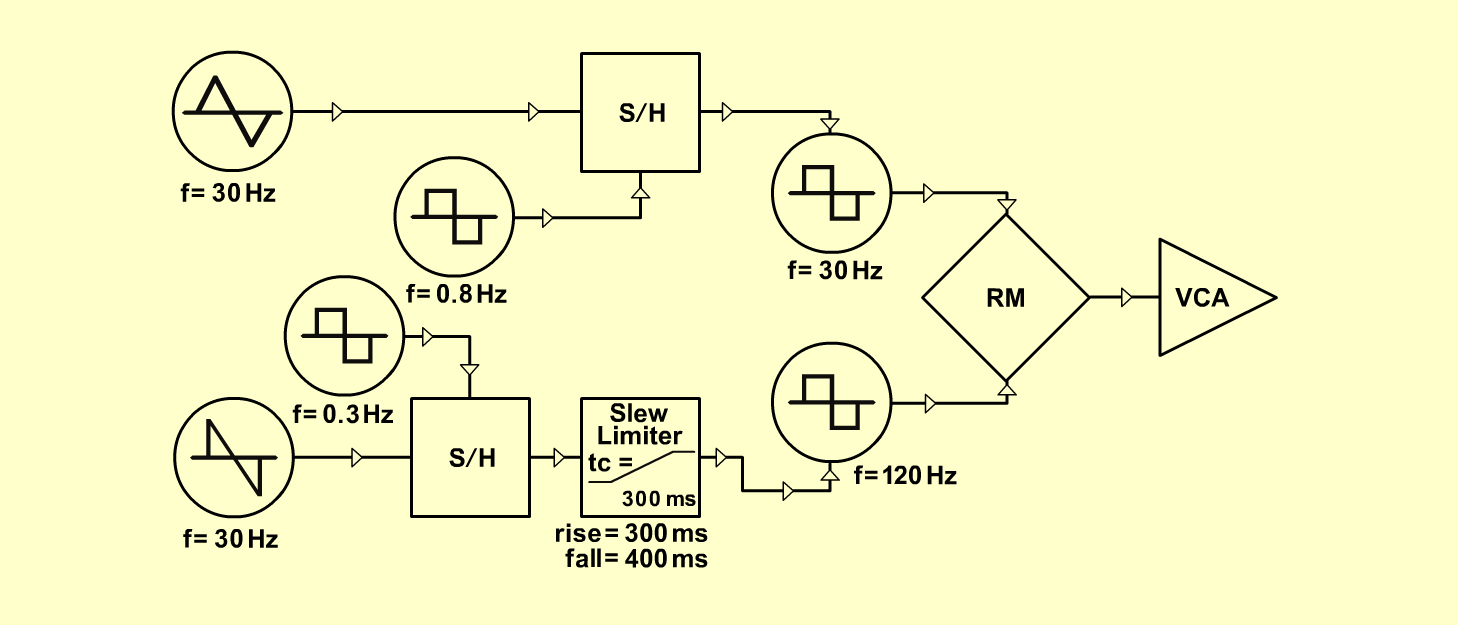Wobble ChaosExperimentalD o e p f e r A - 100 Connections: Settings: A-110/1 (saw) <=> A-148upper (Smp In) A-110/1 (square) <=> A-114 (X In) A-110/1 (tri) <=> A-148lower (Smp In) A-110/2 (square) <=> A-114 (Y In) A-114 (Out) <=> A-131 (Audio In) A-145 (square) <=> A-148upper (Trig In) A-146 (square) <=> A-148lower (Trig In) A-148upper (S&H Out) <=> A-170lower (In) A-148lower (S&H Out) <=> A-110/1 (CV 1) A-170lower (Out) <=> A-110/2 (CV 1) A-131 (Audio Out) <=> Amplifier A-110/1 (Tune = 5, PW = 5, Range = 0) A-110/2 (Tune = 5, PW = 5, Range = +2) A-145 (Frq = 6, Range = L) A-146 (Freq = 10, Wave Form = 5, Range = L) A-170 (Up = 7, Down = 10) Andrew Dalio, January 2003Sound samples Wobble Chaos 1 Wobble Chaos 2